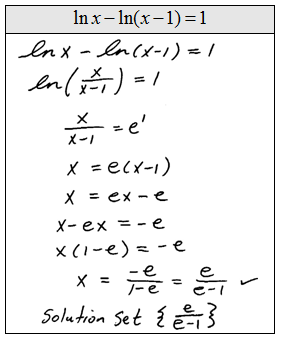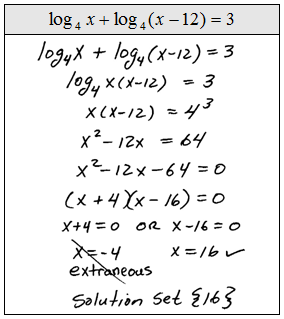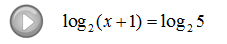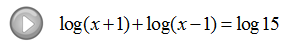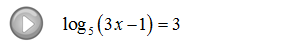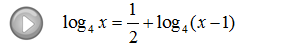## Algebra

Showing posts with label equation. Show all posts
Showing posts with label equation. Show all posts

Now that we have learned how to work with radical expressions, we next move on to solving.

Use caution when solving radical equations because the following steps may lead to extraneous solutions, solutions that do not solve the original equation.

Solve:Step 2: Square both sides of the equation.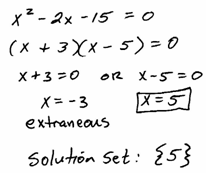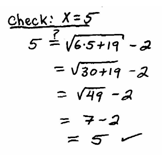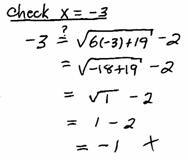Whenever you raise both sides of an equation to an even power, you introduce the possibility of extraneous solutions so the check is essential here.

Solve.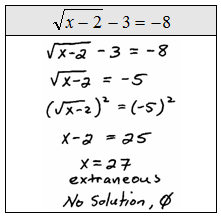The index determines the power to which we raise both sides.  For example, if we have a cube root we will raise both sides to the 3rd power. The property that we are using isfor integers n > 1 and positive real numbers x. After eliminating the radical, we will most likely be left with either a linear or a quadratic equation to solve.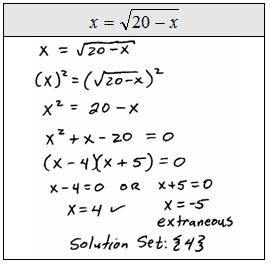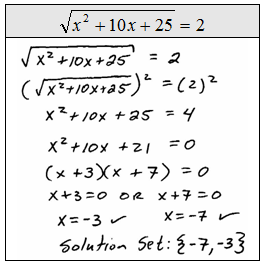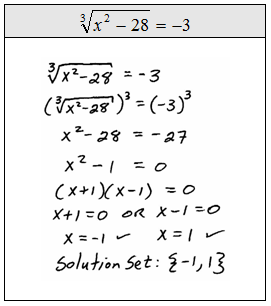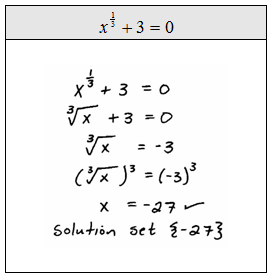The check mark indicates that we have actually checked that the value is a solution to the equation, do not dismiss this step, it is essential.Some radical equations have more than one radical expression.  These require us to isolate each remaining radical expression and raise both sides to the nth power until they are all eliminated.  Be patient with these, go slow and avoid short cuts.
Solve: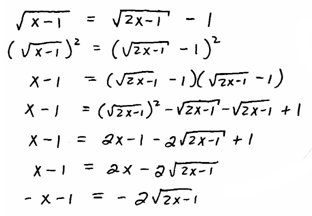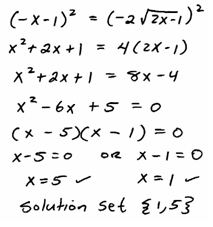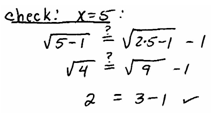Solve: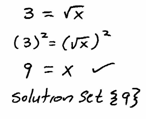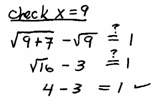Solve: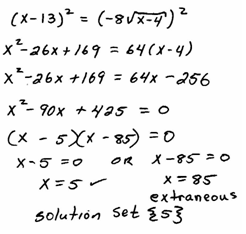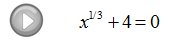### Solving Logarithmic Equations

Use the one-to-one property for logarithms to solve logarithmic equations.

If we are given an equation with a logarithm of the same base on both sides we may simply equate the arguments.

Step 1: Use the rules of exponents to isolate a logarithmic expression (with the same base) on both sides of the equation.
Step 2: Set the arguments equal to each other.
Step 3: Solve the resulting equation.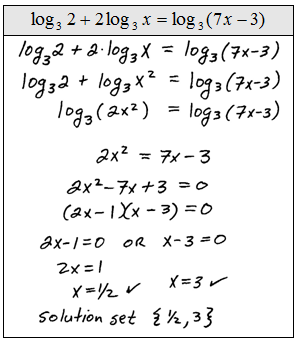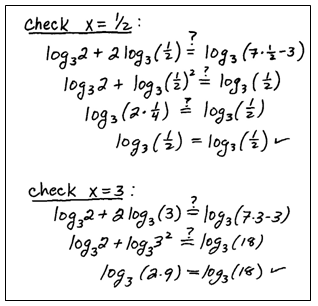Be sure to check to see if the solutions that you obtain solve the original logarithmic equation. In this study guide we will put a check mark next to the solution after we determine that it really does solve the equation. This process sometimes results in extraneous solutions so we must check our answers.
Solve.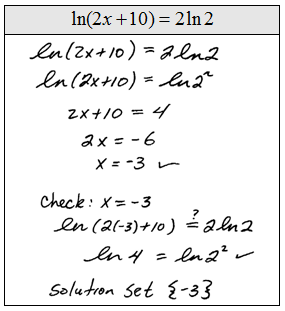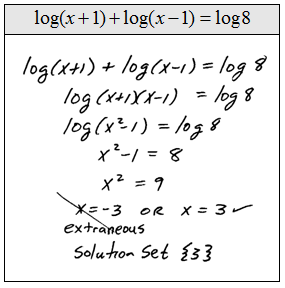Of course, equations like these are very special.  Most of the problems that we will encounter will not have a logarithm on both sides. The steps for solving them follow.

Step 1: Use the properties of the logarithm to isolate the log on one side.
Step 2: Apply the definition of the logarithm and rewrite it as an exponential equation.
Step 3: Solve the resulting equation.If the answer to the logarithmic equation makes the argument negative then it is extraneous.  This does not preclude negative answers. We must be sure to check all of our solutions.

Instructional Video: Solving Logarithmic Equations

Solve.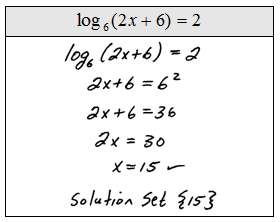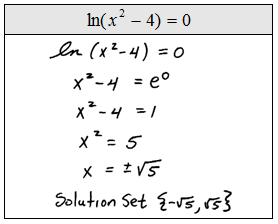Tip:  Not all negative solutions are extraneous!  Look at the previous set of problems and see that some have negative answers. The check mark indicates that we actually plugged the answers in to see that they do indeed solve the original.  Please do not skip this step, extraneous solutions occur often.